Courses

RD Sharma Solutions -Ex-21.2 (Part - 1), Surface Area And Volume Of Sphere, Class 9, Maths Notes | Study RD Sharma Solutions for Class 9 Mathematics - Class 9

Class 9: RD Sharma Solutions -Ex-21.2 (Part - 1), Surface Area And Volume Of Sphere, Class 9, Maths Notes | Study RD Sharma Solutions for Class 9 Mathematics - Class 9

The document RD Sharma Solutions -Ex-21.2 (Part - 1), Surface Area And Volume Of Sphere, Class 9, Maths Notes | Study RD Sharma Solutions for Class 9 Mathematics - Class 9 is a part of the Class 9 Course RD Sharma Solutions for Class 9 Mathematics.
All you need of Class 9 at this link: Class 9

Q1. Find the volume of a sphere whose radius is : (i) 2 cm (ii) 3.5 cm (iii) 10.5 cm.

Sol.

Therefore volume =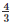πr3

=×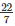×(2)3

= 33.52cm3

Therefore volume =πr3

=××(3.5)3  = 179.666cm3

Therefore volume = 43πr3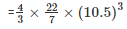= 4851cm3

Q2. Find the volume of a sphere whose diameter is : (i) 14 cm (ii) 3.5 dm (iii) 2.1 m

Sol.

(i)  Diameter = 14cm, Radius(r) =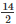= 7cm

Therefore volume =πr3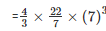= 1437.33cm3

(ii) Diameter = 3.5dm, Radius(r) =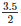= 1.75dm

Therefore volume =πr3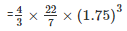= 22.46dm3

(iii) Diameter = 2.1m, Radius(r) =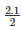= 1.05m

Therefore volume =πr3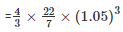= 4.851m3

Q3. A hemispherical tank has the inner radius of 2.8 m.  Find its capacity in liters.

Sol.

Radius of the tank = 2.8m

Therefore Capacity =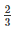πr3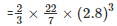= 45.994m3

1m3 = 1000l

Therefore capacity in litres = 45994 litres

Q4. A hemispherical bowl is made of steel 0.25 cm thick. The inside radius of the bowl is 5 cm. Find the volume of steel used in making the bowl.

Sol.

Outer radius = 5 + 0.25 = 5.25

Volume of steel used = Outer volume - Inner volume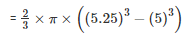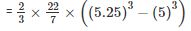= 41.282cm3

Q5. How many bullets can be made out of a cube of lead, whose edge measures 22 cm, each bullet being 2 cm in diameter?

Sol.

Cube edge = 22cm

Therefore volume of the cube =  (22)3  = 10648cm3

And,

Volume of each bullet =πr=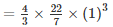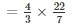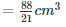Number of bullets =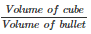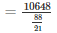= 2541

Sol.

i.e Volume(V1)  =πr3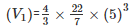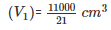i.e Volume(V2)  =πr3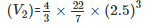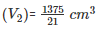Therefore number of laddoos =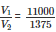= 8

Q7. A spherical ball of lead 3 cm in diameter is melted and recast into three spherical balls. If the diameters of two balls be 32cm and 2 cm, find the diameter of the third ball.

Sol.

Volume of lead ball =πr3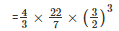Diameter of first balld =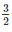cm

Radius of first ball r1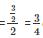cm

Diameter of second ball d2 = 2cm

Radius of second ball r2  =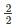cm = 1cm

Diameter of third balld3  = d

Radius of third ball r3  =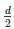cm

Volume of lead ball =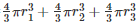Volume of lead ball =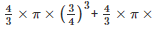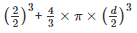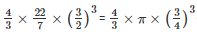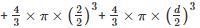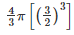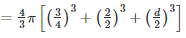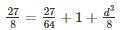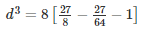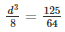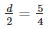d =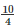d = 2.5cm

Q8. A sphere of radius 5 cm is immersed in water filled in a cylinder, the level of water rises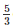cm. Find the radius of the cylinder.

Sol.

Volume of sphere =πr3

=×π×(5)3

Height of water rised =cm

Volume of water rised in cylinder = πr2h

Therefore, Volume of water rises in cylinder = Volume of sphere

Let r be the radius of the cylinder

πr2h =πr3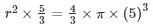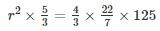r2 = 20×5

r =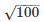r = 10cm

Q9. If the radius of a sphere is doubled, what is the ratio of the volume of the first sphere to that of the second sphere?

Sol.

Let v1 and v2 be the volumes of the first and second sphere respectively

Radius of the first sphere = r

Radius of the second sphere = 2r

Therefore,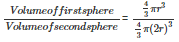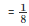Q10. A cone and a hemisphere have equal bases and equal volumes. Find the ratio of their heights.

Sol.

Given that

Volume of the cone = Volume of the hemisphere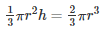r2h = 2r3

h = 2r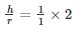= 2

Therefore

Ratio of their heights = 2 : 1

Q11. A vessel in the form of a hemispherical bowl is full of water. Its contents are emptied in a right circular cylinder. The internal radii of the bowl and the cylinder are 3.5 cm and 7 cm respectively. Find the height to which the water will rise in the cylinder.

Sol.

Given that

Volume of water in the hemispherical bowl = Volume of water in the cylinder

Let h be the height to which water rises in the cylinder

Inner radii of the bowl =  r = 3.5cm

Inner radii of the bowl =  r2  = 7cm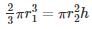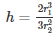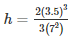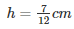Q12. A cylinder whose height is two thirds of its diameter has the same volume as a sphere of radius 4 cm. Calculate the radius of the base of the cylinder.

Sol.

Given that

Height of the cylinder =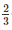diameter

We know that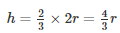Volume of the cylinder = Volume of the sphere

πr2h =πr3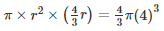(r)3 = (4)3

r = 4cm

Q13. A vessel in the form of a hemispherical bowl is full of water. The contents are emptied into a cylinder. The internal radii of the bowl and cylinder are respectively 6 cm and 4 cm. Find the height of water in the cylinder.

Sol.

It is given that

Volume of water in hemispherical bowl = Volume of cylinder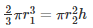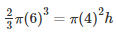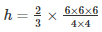h = 9cm

Q14. A cylindrical tub of radius 16 cm contains water to a depth of 30 cm. A spherical iron ball is dropped into the tub and thus level of water is raised by 9 cm. What is the radius of the ball?

Sol.

Let r be the radius of the iron ball

Radius of the cylinder = 16cm

Then,

Volume of iron ball = Volume of water raised in the hub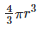= πr2h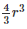= (16)2×9

r=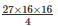r3 = 1728

r = 12cm

Therefore radius of the ball = 12cm.

Q15. A cylinder of radius 12 cm contains water to a depth of 20 cm. A spherical iron ball is dropped into the cylinder and thus the level of water is raised by 6.75 cm. Find the radius of the ball. (Use =).

Sol.

Given that :

Radius of the cylinder = 12cm =  r1

Raised in  raised = 6.75 cm =  r2

Volume of water raised = Volume of the sphere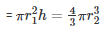=  12×12×6.75 =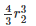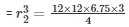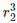= 729

=  r2  = 9cm

Radius of the sphere is 9cm

Q16. The diameter of a copper sphere is 18 cm. The sphere is melted and is drawn into a long wire of uniform circular cross - section. If the length of the wire is 108 m, find its diameter.

Sol.

Given that diameter of a copper sphere = 18cm

Radius of the sphere = 9cm

Length of the wire = 108m = 10800cm

Volume of cylinder = Volume of sphere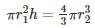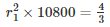×9×9×9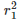= 0.009

r1 = 0.3cm

Therefore Diameter = 2×0.3 = 0.6cm

The document RD Sharma Solutions -Ex-21.2 (Part - 1), Surface Area And Volume Of Sphere, Class 9, Maths Notes | Study RD Sharma Solutions for Class 9 Mathematics - Class 9 is a part of the Class 9 Course RD Sharma Solutions for Class 9 Mathematics.
All you need of Class 9 at this link: Class 9Use Code STAYHOME200 and get INR 200 additional OFF Use Coupon Code

Top Courses for Class 9RD Sharma Solutions for Class 9 Mathematics

91 docs

Top Courses for Class 9Track your progress, build streaks, highlight & save important lessons and more!

,

,

,

,

,

,

,

,

,

,

,

,

,

,

,

,

,

,

,

,

,

,

,

,

,

,

,

,

,

,

;